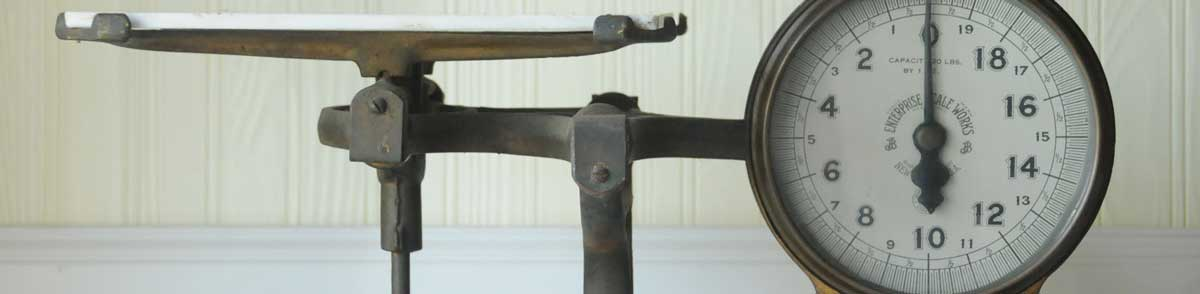# Calories to Ounces Conversion

Enter the weight in calories below to get the value converted to ounces.

Results in Ounces:1 kcal = 0.004571 oz
Do you want to convert ounces to calories?

## How to Convert Calories to OuncesTo convert a calorie measurement to an ounce measurement, multiply the weight by the conversion ratio.

Since one calorie is equal to 0.004571 ounces, you can use this simple formula to convert:

ounces = calories × 0.004571

The weight in ounces is equal to the calories multiplied by 0.004571.

For example, here's how to convert 500 calories to ounces using the formula above.
500 kcal = (500 × 0.004571) = 2.285714 oz

Calories are a unit of energy, and ounces are a unit of weight. Keep reading to learn more about each unit of measure.

## Calories

Converting calories is actually a conversion of energy burned by the body performing work rather than actual weight. When measuring the energy content in foods, the large calorie, or kilocalorie, is used, but it's often still just called a "calorie."

One calorie (large calorie) is equal to the heat needed to raise the temperature of one kilogram of water by 1 degree Celsius. You'll need to burn 3,500 calories more than you eat to lose one pound of weight.

Calories can be abbreviated as kcal; for example, 1 calorie can be written as 1 kcal.

## Ounces

One ounce is a unit of mass/weight equal to 1/16 of a pound. The common ounce should not be confused with the troy ounce, which is equal to 1/12 of a troy pound.

The ounce is a US customary and imperial unit of weight. Ounces can be abbreviated as oz; for example, 1 ounce can be written as 1 oz.

## Calorie to Ounce Conversion Table

Calorie measurements converted to ounces
Calories Ounces
1 kcal 0.004571 oz
2 kcal 0.009143 oz
3 kcal 0.013714 oz
4 kcal 0.018286 oz
5 kcal 0.022857 oz
6 kcal 0.027429 oz
7 kcal 0.032 oz
8 kcal 0.036571 oz
9 kcal 0.041143 oz
10 kcal 0.045714 oz
20 kcal 0.091429 oz
30 kcal 0.137143 oz
40 kcal 0.182857 oz
50 kcal 0.228571 oz
60 kcal 0.274286 oz
70 kcal 0.32 oz
80 kcal 0.365714 oz
90 kcal 0.411429 oz
100 kcal 0.457143 oz
200 kcal 0.914286 oz
300 kcal 1.3714 oz
400 kcal 1.8286 oz
500 kcal 2.2857 oz
600 kcal 2.7429 oz
700 kcal 3.2 oz
800 kcal 3.6571 oz
900 kcal 4.1143 oz
1,000 kcal 4.5714 oz

## References

1. Brazier, Yvette, How many calories do you need?, Medical News Today, https://www.medicalnewstoday.com/articles/263028
2. U.S. Department of Agriculture, I want to lose a pound of weight. How many calories do I need to burn?, https://www.nal.usda.gov/fnic/i-want-lose-pound-weight-how-many-calories-do-i-need-burn
3. James Bucki, Troy Ounce vs. Ounce, The Spruce, https://www.thesprucecrafts.com/troy-ounce-vs-ounce-4588158